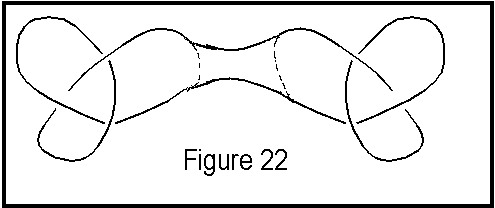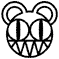# KNOT COMPOSITIONAdams does a nice job of explaining how knots can be composed.  He describes composition of knots like this: a new knot can be made from two other knots, called knot J and knot K, by taking each knot projection, removing a small arc from each, and connecting the four endpoints, as shown in figure 22.  The two arcs that are chosen are assumed to not overlap and not have crossings between themselves or the original knot projections.See figure 23 for an example of how NOT to compose two knots. The result if done correctly is called the composition of the two knots.  Adams denotes the composition as J#K. 

Just like in the integers, a knot K is called composite if two nontrivial knots J1 and J2 (that is, knots that are not the unknot) exist which, when composed, produce K.  J1 and J2 are called the factor knots.  As you can easily guess, a prime knot only has as factors itself and the unknot.  See figure 24 for some examples of prime knots.A rather interesting point Adams makes is that if the unknot happens to be composite, then every knot must be composite.  It may seem obvious at first thought that the unknot is not composite, but figure 25 shows an unknot that looks as if it could be composite.  If the unknot is composite, then it must be made up of two nontrivial knots.  That is, every knot which is the composition of itself with the unknot would really be a composition of two nontrivial knots, and hence be composite.  So every knot would be composite.  However, Adams proves that this is not true, just as the analogous situation in the integers is not true, where 1 is not a composite number. 

I make one last point by noting that this analogy between composites and primes in the integers and in knots continues by noting the theorem stating the uniqueness and existence of a decomposition of knots by Murasugi:

(1) Any knot can be decomposed into a finite number of prime knots.
(2) This decomposition, excluding the order, is unique.  That is, suppose we can decompose K in two ways: K1, K2, ..., Km, and K1`, K2`, ..., Kn`.  Then n = m, and, furthermore, if we suitably choose the numbering of K1, K2, ..., Km, then K1 = K1`, ..., Kn = Kn`. 
A proof of this is very involved and can be found in Burde and Kieschang's book.kMain menu      1. History              2.Intro               3.Invariants      4.Composition   5.Conclusion